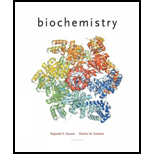# Answers to all problems are at the end of this book. Detailed solutions are available in the Student Solutions Manual, Study Guide, and Problems Book. Calculate the Free Energy Change for Synthesis of a Polynucleotide (Integrals with Chapter 3.) The synthesis of RNA can be summarized by the reaction: n NTP → ( NTP ) n + n PP i What is the △ GÂ°â€™ overall far synthesis of an RNA molecule 100 nucleotides in length, assuming that the △ GÂ°â€™ for transfer of an NMP from an NTP to the 3'-O of polynucleotide chain is the same as the △ G' for Transfer of an NMP from an NTP to H 2 O (Use data given in Table 3.3.)### Biochemistry

6th Edition
Reginald H. Garrett + 1 other
Publisher: Cengage Learning
ISBN: 9781305577206

#### Solutions

Chapter
Section### Biochemistry

6th Edition
Reginald H. Garrett + 1 other
Publisher: Cengage Learning
ISBN: 9781305577206
Chapter 10, Problem 10P
Textbook Problem
13 views

## Answers to all problems are at the end of this book. Detailed solutions are available in the Student Solutions Manual, Study Guide, and Problems Book. Calculate the Free Energy Change for Synthesis of a Polynucleotide (Integrals with Chapter 3.) The synthesis of RNA can be summarized by the reaction: n NTP → ( NTP ) n + n PP i What is the △ GÂ°â€™overall far synthesis of an RNA molecule 100 nucleotides in length, assuming that the △ GÂ°â€™ for transfer of an NMP from an NTP to the 3'-O of polynucleotide chain is the same as the △ G' for Transfer of an NMP from an NTP to H2O (Use data given in Table 3.3.)

Interpretation Introduction

Interpretation:

The value of ΔG°'overall for the synthesis of an RNA molecule 100 nucleotides in length is to be calculated.

Concept introduction:

The RNA consists of four base pairs. Four base pairs of RNA molecule are adenine, uracil, guanine and cytosine. The base adenine is complementary with uracil and guanine is complementary with cytosine.

### Explanation of Solution

The given reaction for the synthesis of RNA molecule is,

nNTP(NMP)n+nPPi

It is given that ΔG°' for the transfer of a NMP from a NTP to the 3'O of polynucleotide chain is the same as the ΔG°' for transfer of a NMP from a NTP to H2O .

The given standard value of ΔG°' for the transfer of a NMP from a NTP to the 3'O of polynucleotide chain is 32.3kJ/mol .

The RNA is 100 nucleotides in length must requires to transfer at least 99 NMPs from NTP to the 3'O of polynucleotide chain

### Still sussing out bartleby?

Check out a sample textbook solution.

See a sample solution

#### The Solution to Your Study Problems

Bartleby provides explanations to thousands of textbook problems written by our experts, many with advanced degrees!

Get Started

Find more solutions based on key concepts
A way to prevent spoilage of unsaturated fats and make them harder is to change their fatty acids chemically th...

Nutrition: Concepts and Controversies - Standalone book (MindTap Course List)

1.34 Convert the value 0.120 ppb into ppm.

Chemistry for Engineering Students

Explain why certain carbohydrates are called sugars.

Chemistry for Today: General, Organic, and Biochemistry